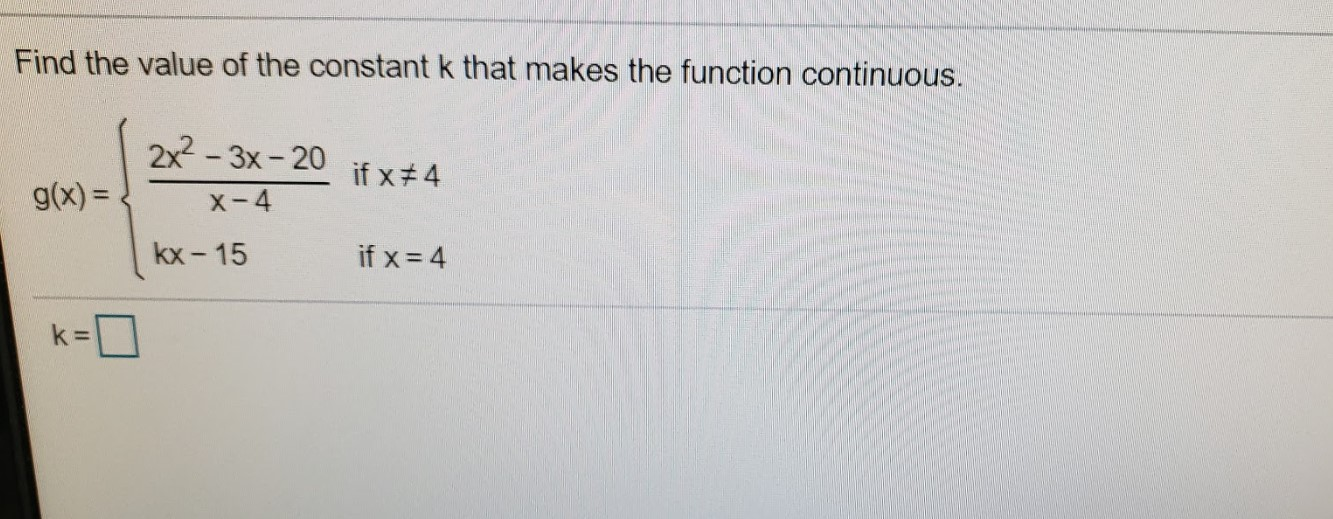# Find the value of the constant k that makes the function continuous.2x - 3x- 20if x#4g(x) =x-4kx - 15if x = 4

Question
6 viewshelp_outlineImage TranscriptioncloseFind the value of the constant k that makes the function continuous. 2x - 3x- 20 if x#4 g(x) = x-4 kx - 15 if x = 4 fullscreen
check_circle

star
star
star
star
star
1 Rating
Step 1

A function f(x) is continuous at a point x = a iff:

Step 2

Here the given function g(x) is continuous at x = 4. therefo...

### Want to see the full answer?

See Solution

#### Want to see this answer and more?

Solutions are written by subject experts who are available 24/7. Questions are typically answered within 1 hour.*

See Solution
*Response times may vary by subject and question.
Tagged in

### Calculus